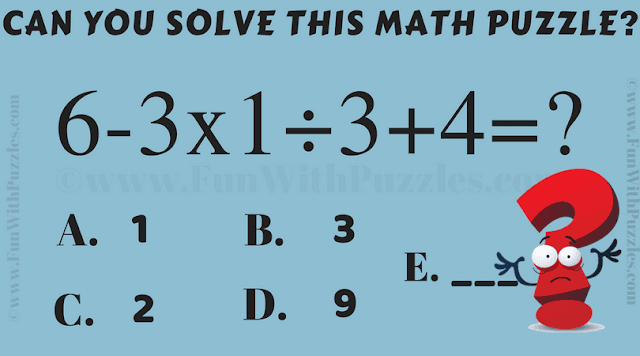This a very interesting maths riddle for school going students which will test your mathematical skills. When we grow up we tend to forget the basics. This is one of the maths riddles which will test the knowledge of your mathematical skills. Let us see if you can answer this simple math riddle correct. If your answer is to not correct then this is time to revise your mathematical skills :).Can you solve this Maths Riddle?
The answer to this "Maths Riddle for Teens", can be viewed by clicking on the answer button.